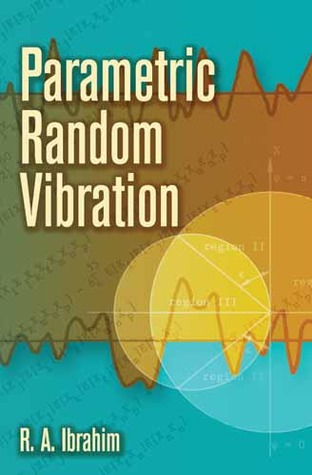Home » Parametric Random Vibration by Raouf A. Ibrahim# Parametric Random Vibration

## Raouf A. Ibrahim

Published January 11th 2008
ISBN : 9780486462622
Paperback
352 pages
Book Rating:Enter the sum

 About the Book Coherent and systematic, this text explores the behavior of  linear and nonlinear dynamical systems subject to parametric random vibrations, by means of the theory of stochastic processes, stochastic differential equations, and applied dynamics. It distills decades of research to formulate new stochastic stability theorems and analytical techniques for determining the random response of nonlinear systems. In addition, it resolves controversies and paradoxes related to the interpretation of certain stochastic processes and the use of analytical methods.After a brief overview of parametric vibration and its subclasses, the text surveys the fundamental concepts of random processes and their calculus rules, emphasizing the main elements necessary for the analysis of parametric vibration problems. Subsequent chapters address the stability and response analyses of linear and nonlinear systems with random coefficients. The final chapter presents an extensive compilation of experimental results from the literature of the field.